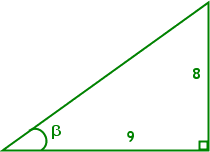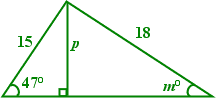Inverses of Trigonometric RatiosYou've learned how to use trig ratios to solve right triangles, finding the lengths of the sides of triangles. But what if you have the sides, and need to find the angles? You know that you can take side lengths and find trig ratios, and you know you can find trig ratios (in your calculator) for angles. What is missing is a way to go from the ratios back to the original angles. And that is what "inverse trig" values are all about.

If you look at your calculator, you should see, right above the "SIN", "COS", "TAN" buttons, notations along the lines of "SIN–1", "COS–1", and "TAN–1", or possibly "ASIN", "ACOS", and "ATAN". These are what you'll use to find angles from ratios.

The first set of notations, with the "minus one" exponent, lists the inverse sine, the inverse cosine, and the inverse tangent. The second set of notations, with the "A" before each name, lists the arcsine, arccosine, and arctangent. These are two notations for exactly the same thing.

Grab your calculator, and take the sine of some angle value between zero and ninety degrees. Whatever result you get, do the inverse sine ("SIN–1") or the arcsine ("ASIN") of that value, and you should get the value you started with. That's what the inverses of trig ratios do: they give you the angle that goes with that trig ratio.

• Find the measure of angle α, to the nearest degree.
•They've given me the opposite side from α and the hypotenuse, so I can form the sine ratio:

9/10 = sin(α) = 0.9

Plugging 0.9 into SIN–1 in my calculator, I get α = 64.15806724...

m(α) = 64°

 Find the measure of angle β, accurate to one decimal place. They've given me the lengths of the side opposite β (being 8) and the side adjacent to β (being 9). Since the tangent is opposite over adjacent, I can form the tangent ratio with what they've given me: 8/9 = tan(β)I won't use the decimal for 8/9, because that could introduce round-off error. Instead, I'll work with the "exact" fraction, and plug TAN–1(8/9) into my calculator directly. The result is β = 41.63353934.... m(β) = 41.6°

• Find the length of side p and the measure of angle m, as shown on the diagram. Give each answer correct to the nearest whole number or degree.How on earth am I supposed to find m and p when I only have one number for that triangle? All I have is the hypotenuse! Oh, wait... Copyright © Elizabeth Stapel 2010-2011 All Rights Reserved

I can use the angle and hypotenuse on the left-hand triangle to find the height p, and this will give me two numbers for the right-hand triangle. With that, I can find m.

The left-hand triangle has "opposite", hypotenuse, and angle, so I'll work with the sine ratio:

p/15 = sin(47°)
p = 15×sin(47°) = 10.97030552...

Now that I know p = 11, I can find the measure of angle m:

11/18 = sin(m°)
sin–1(11/18) = m° = 37.66988696...

p = 11 and m° = 38°

• A 5-m ladder is leaning against a building, with the base of the ladder being two meters from the side of the building. What angle does the ladder form with the ground?

 As usual, I start with a picture. It doesn't need to be "exact" or "to scale"; I just need enough of a picture to be able to keep track of what I'm doing. With respect to the angle they want me to find, I have "adjacent" and the hypotenuse, so I'll use the cosine ratio. 2/5 = cos(θ) cos–1(2/5) = θ = 66.42182152... The ladder forms an angle of about 66.4°.Any time you have two sides of a triangle and need an angle, figure out the trig ratio that uses those two sides, and use the appropriate inverse button to find the angle that goes with that ratio. And remember to put the "degree" sign on your answer.